# Lies, Damned Lies, and Statistics

• @J.Hilk
The question posed is: "Given that your result is reported as positive, what is the probability that you actually do have the disease?"

Are you claiming that the answer to that is your "1.1%"? I say it's ~ 1 in 11, more like "9.09%".

well it is 0.099 % you have it and it is diagnosed
to 0.999 % you don't have it and it is diagnosed

=> ~10% chance you actually have it, when it is diagnosed ?

• @J.Hilk
Well, your "~ 10%" is not far off my "~ 9.1%", so we're close, though I'll stick (as per my black-balls-in-bag) to my 9.1% being closer than 10%.

BTW, your:

well it is 0.099 % you have it and it is diagnosed

is slightly off. We know (before the test) there is a 0.1% chance you have the ailment, and that the test "correctly diagnoses all [actual] cases". So, depending on your phrasing, this should remain at 0.1%. The bit where you originally wrote:

you have it 0.001 and the test shows it 0.99 => 0.00099

you have it 0.001 and the test shows it 1.0 => 0.001

Then you have:

The test always has a result, but there's a 1 % chance the result is the exact opposite.

Not quite. It does not do "the exact opposite". There is a 1% chance it reports positive when it should be negative. But the opposite is not the case: it does not report negative when it should be positive ever.

• There is a 0.01% I have the disease, in which case I will deffo be told I do.
• There is a 0.1% I don't have the disease, but will be told I do.
• [Note that the above 2 cases are mutually exclusive, with no dependencies.]
• The test will report 0.11% total positives. 11 people out of 1,000. 10 will be incorrect, 1 will be correct. You have a 1 in 11 chance of being the positive one, and a 10 in 11 chance of being one of the false ones. Period.

• JonB nailed it. His analysis was spot-on, with one minor nit: The problem stated:

Someone devises a test for this disorder which, in correctly diagnoses all cases, but also reports a false positive exactly 1% of the time. As stated, the false positive rate is given without regard to any true positives -- it occurs at a rate of 1%. In a population, it will "lie" about 10 individuals. So, the correct answer is exactly (not nearly) 1 in 11.

Regarding the "everything is 50-50" assertion: this has its roots in philosophy as much as it does in probability, but it's still valid IMO. I'd love to hear how Mike Caro
would respond to your interesting point.

• I like algebra, so here's some notation from probability theory:

• P(A): Probability that A occurs
• P(A ∩ B): Probability that A and B both occur
• P(A | B): Probability that A occurs, given that B occurs

In this example,

• A: Have the disease
• B: Get a positive test result
• Out of every 1,000 people, 1 has the affliction.

`P(A) = 0.001`

• The test will always identify that one person as being afflicted.

`P(B | A) = 1`

By the axiom of probability, P(B∩A) = P(B|A) * P(A) so `P(B ∩ A) = 0.001`

• Additionally, the test will report 10* other people as being afflicted who in fact are healthy.

[* Actually, the remaining population is 999, so really 9.99 rather than 10.0. This would affect my final figure, but I imagine you're not looking for that degree of accuracy, so my answer will be right to nearest couple of decimal places!]

`P(B | ¬A) = 0.01`

Similarly to before, `P(B ∩ ¬A) = 0.00999`

Well, in this case, the test has reported 11 people as positive. 1 is genuinely positive, while 10 are false positive.

P(B) = P(B ∩ A) + P(B ∩ ¬A) so `P(B) = 0.01099`

My conclusion:

• Before the test result I had 1 in 1,000 chance of the terminal illness you are imposing.

Yep, before the test, nothing was given, so you can only use P(A). We already know `P(A) = 0.001`.

• After the test I have a 1 in 11 chance of being the positive one, and a 10 in 11 chance of being one of the falsies.

Given that you got a positive test result, what is the probability that you have the disease?

P(A | B) = P(A ∩ B) / P(B) = 0.001 / 0.01099 so `P(A | B) = 0.0909918...` which is a teensy bit more than 1 in 11.

If it helps any, you can also think of this as balls in a bag:

• There is 1 black ball, which has "You're toast" on a piece of paper inside it.
• There are 10 black balls, which have "Only kidding" on a piece of paper inside them.
• There are 989 white balls.

You put your hand in the bag and pull out a ball. It's black :( Given that, until you open the ball and look at the piece of paper, there's a 1 in 11 chance it contains the fateful news.

Right?

Haha, awesome analogy!

As Mike Caro (a brilliant professional gambler) has observed, "in the beginning, everything was even money." In other words, lacking any other information, one's best guess as to the probability of ANYTHING is 50-50.

I don't know if there was a context in which he wrote this which you have omitted, but that's a very strange statement. Lacking any information at all, one's "best guess" of a probability should not be anything like "50-50". I can only think a gambler might think that way!

I don't think that "50-50" means "Each answer has a 50% chance to be correct". Rather, it means "Each answer has the same chance of being correct as every other possible answer".

So, if you're guessing heads or tails, there are only 2 possible answers so you have a 50% chance of getting it right. However, with the bag of balls, if the bag is big enough to hold 99 balls then there are 100 possible answers, so you have a 1% chance of getting it right.

• JKSH got it right as well (with the same very minor glitch as JonB).

well it is 0.099 % you have it and it is diagnosed

Actually 0.1% (as discussed above).

to 0.999 % you don't have it and it is diagnosed

1%.

=> ~10% chance you actually have it, when it is diagnosed ?

10 false positives, one real positive: your chances are 1 in 11, or about 9%. You were pretty close.

• Since you guys did so well on that one, here's another: I hand you a bag, inside which are three coins. The coins appear identical, but while two are "fair," one will always land heads-up.

You pull a coin from the bag, and toss it three times. You get a head every time. What are the chances you pulled the unfair coin?

(Those who get this right might be ready for the extremely unintuitive Monte Hall problem...)

• @JKSH
Two quick observations:

P(A | B) = P(A ∩ B) / P(B) = 0.001 / 0.01099 so P(A | B) = 0.0909918... which is a teensy bit more than 1 in 11.

There is still something wrong here with where you go about calculating these figures, but I'm too tired to spot it. @mzimmers said of my solution above:

His analysis was spot-on, with one minor nit:
[...]
So, the correct answer is exactly (not nearly) 1 in 11.

In my first attempt, at the end I stated:

After the test I have a 1 in 11 chance of being the positive one, and a 10 in 11 chance of being one of the falsies.

And in my second clarification earlier, I had come to the same conclusion when I wrote:

The test will report 0.11% total positives. 11 people out of 1,000. 10 will be incorrect, 1 will be correct. You have a 1 in 11 chance of being the positive one, and a 10 in 11 chance of being one of the false ones. Period.

That was my attempt to say ("Period") that I had realized my previous talk about "999" & "roundings" was unnecessary & inaccurate. Like @mzimmers I conclude the chance is exactly 1 in 11.

I don't think that "50-50" means "Each answer has a 50% chance to be correct". Rather, it means "Each answer has the same chance of being correct as every other possible answer".

The second sentence might be a better way of phrasing it. Which, certainly to my mind/understanding, should never be referred to as "50-50".

• (Those who get this right might be ready for the extremely unintuitive Monte Hall problem...)

Darn, I was going to quote that one! :) (If you do, I won't say a word, till it's solved by someone who doesn't know.)

P.S.
Are you old enough to have watched the show live in the USA? ;-)

• You pull a coin from the bag, and toss it three times. You get a head every time. What are the chances you pulled the unfair coin?

``````8 in 10
``````

• You pull a coin from the bag, and toss it three times. You get a head every time. What are the chances you pulled the unfair coin?

``````8 in 10
``````

Correct (though I would have said 4 in 5). Care to share with the other students how you arrived at this answer?

• @mzimmers
I chose to write "8 in 10" rather than "4 in 5" deliberately, because of the way I reached the figure mentally.

I thought I would not explain, at least for now, so that others might have their opportunity to think it through and see what they came up with. Like you did for the other one, perhaps I should wait for 24 hours before explaining! BTW, I found this one easier to think through than the first one, for some reason --- perhaps because the other one gave me medical frights? ;-)

• Hah...fair enough, though I'm now curious as to how you ended up at 8 in 10...but if everyone else can can wait for the answer, I suppose I can wait for the explanation.

• @mzimmers I'll post over the weekend... :) Probably only you & I care now!

• JonB nailed it. His analysis was spot-on, with one minor nit: The problem stated:

Someone devises a test for this disorder which, in correctly diagnoses all cases, but also reports a false positive exactly 1% of the time. As stated, the false positive rate is given without regard to any true positives -- it occurs at a rate of 1%. In a population, it will "lie" about 10 individuals.

So you meant "1% of the whole population receives a false positive" (`P(B ∩ ¬A) = 0.01`).

I thought you meant "1% of the healthy people receive a false positive" (`P(B | ¬A) = 0.01`).

P(A | B) = P(A ∩ B) / P(B) = 0.001 / 0.01099 so P(A | B) = 0.0909918... which is a teensy bit more than 1 in 11.

There is still something wrong here with where you go about calculating these figures, but I'm too tired to spot it.

It boiled down to the interpretation of the false-positive rate (see above). With the correct interpretation, we have:

• `P(A) = 0.001` (0.1% of the population have the disorder)
• `P(B | A) = 1` (The test detects the disorder 100% of the time)
• `P(B ∩ ¬A) = 0.01` (The test has a 1% false positive rate within the whole population)

Finding intermediate parameters,

• P(B∩A) = P(B|A) * P(A)`P(B ∩ A) = 0.001` (0.1% of the whole population have the disorder AND get a positive result)
• P(B) = P(B∩A) + P(B ∩ ¬A)`P(B) = 0.011` (1.1% of the whole population get a positive test result)

Finally,

• P(A | B) = P(A∩B) / P(B)`P(A | B) = 1/11` (Given that I got a positive result, I have 1 in 11 chance of having the disorder)

All good! :-D

Since you guys did so well on that one, here's another: I hand you a bag, inside which are three coins. The coins appear identical, but while two are "fair," one will always land heads-up.

You pull a coin from the bag, and toss it three times. You get a head every time. What are the chances you pulled the unfair coin?

I used the same method as my first attempt. Same equations, just different starting numbers.

`P(X|Y) = 0.8` where

• X: Got the unfair coin
• Y: Flipped 3 times and got 3 heads

P.S. Thanks for the fun puzzles, @mzimmers! I used to do them in school/university but haven't done any in a while.

• @mzimmers

I don't use @JKSH 's equations --- too much brainache!

The method is just:

• There are 8 permutations from flipping a coin 3 times.
• The unfair coin produces 3 heads in all of its permutations.
• The fair coins each produce 1 set of 3 heads in each of theirs.
• Thus of the possible 24 outcomes, there are 10 with all heads, and of those 2 are produced by the fair coins while 8 are produced by the weighted one.

Hence my initial writing of `8 in 10`, rather than simplifying :)

You should probably now throw Monte Hall at @JKSH :)

• @JonB

Well done, and well presented. When I was faced with this problem, I did it slightly differently (1/3 * 100%) vs. (2/3 * 12.5%). The underlying logic is the same.

JKSH's notations are just a formal representation of what we're doing. Given that I took my only statistics class nearly 40 years ago, I've forgotten all the notation, though I remember most of the principles. As long as we all get to the right answers, the various approaches are equally valid.

I'll bring up Monte Hall if KJSH chimes in. And yes, I can remember watching that show live...good entertainment (if you're 12 years old).

• @mzimmers

Given that I took my only statistics class nearly 40 years ago, I've forgotten all the notation, though I remember most of the principles

In that case, please remind me what the "Chi squared" test thingy is? I remember the teacher banging on about that one. And no, you are not allowed to look it up. :)

• Chi squared...ew.

"Math's hard; let's go shopping!" (Barbie from the pre men-are-pigs era)

• "Math's hard; let's go shopping!" (Barbie from the pre men-are-pigs era)

LOL.

• I don't use @JKSH 's equations --- too much brainache!

I do find verbal descriptions more meaningful and intuitive, but I also find equations more systematic and comprehensive.

Descriptions help me to understand the "reality" of a problem, while equations help me to see connections and patterns (either within the same problem, or across different problems)

@JonB I've taken the liberty of translating English into Equations :-) (Your statements in bold)

• X: Got the unfair coin
• Y: Flipped 3 times and got 3 heads

Starting info:

• `P(X) = 1/3` (I have a 1 in 3 chance of getting the unfair coin)
• `P(Y | X) = 1` (The unfair coin produces 3 heads in all of its permutations / Given that I got the unfair coin, I'm guaranteed to flip 3 heads in a row)
• `P(Y | ¬X) = 1/8` (There are 8 permutations from flipping a coin 3 times. The fair coins each produce 1 set of 3 heads in each of theirs. / Given that I didn't get the unfair coin, I have a 1 in 2^3 chance of flipping 3 heads in a row)

Intermediate parameters:

• P(¬X) = 1 - P(X)`P(¬X) = 2/3` (I have a 2 in 3 chance of getting a fair coin)
• P(Y ∩ X) = P(Y|X) * P(X)`P(Y ∩ X) = 1/3` (I have a 1 in 3 chance of getting the unfair coin AND flipping 3 heads in a row)
• P(Y ∩ ¬X) = P(Y|¬X) * P(¬X)`P(Y ∩ ¬X) = 1/12` (I have a 1 in 12 chance of getting a fair coin AND flipping 3 heads in a row)
• P(Y) = P(Y|X) + P(Y|¬X)`P(Y) = 5/12` (of the possible 24 outcomes, there are 10 with all heads)

Finally:

• P(X | Y) = P(X ∩ Y) / P(Y)`P(X | Y) = 4/5` (...[of these 10,] 8 are produced by the weighted [coin]. / Given that I flipped 3 heads in a row, there is a 4 in 5 chance that I have the unfair coin)

You should probably now throw Monte Hall at @JKSH :)

Sorry, I looked up the Wikipedia article when it was first mentioned here!

In that case, please remind me what the "Chi squared" test thingy is? I remember the teacher banging on about that one. And no, you are not allowed to look it up. :)

I don't remember how to use it anymore, but I remember using it lots in biology class to test for mutations in a population.

"Math's hard; let's go shopping!" (Barbie from the pre men-are-pigs era)

For me, shopping is hard. Too many choices; need to guard against marketers' tactics; need to research to find a good deal; need to haggle or negotiate...

...let's do math! It's just me, my comfy chair, and my trusty pen+paper.

• In that case, please remind me what the "Chi squared" test thingy is?

You pose a hypothesis (e.g you have a model of something) and you want to test how well your model fits the experimental data you have - you calculate the χ squared and you get your answer. There's a lot of theory behind it, but you can think of it in simple terms as the (quadratic) measure of the population's dispersion around your model - i.e. how far the real population is from the modelled population.

• @mzimmers said in Lies, Damned Lies, and Statistics:

"Math's hard; let's go shopping!" (Barbie from the pre men-are-pigs era)

For me, shopping is hard. Too many choices; need to guard against marketers' tactics; need to research to find a good deal; need to haggle or negotiate...

In that case, you don't seem to have a woman. If you did, I would expect her to insist on making all the shopping choices on your behalf, so it wouldn't be an issue... ;-)
["JB: Unreconstructed from the pre-PC era."]

• @kshegunov

1. So that's a rather different thing from standard deviation, right. So you make a model, calculate with it, then discover how inaccurate it is by going back and examining the real population, and then make something squared out of it. Is that it?

2. Bonus point for finding the χ key on your keyboard.

While I'm at it.... The other thing I remember the teach banging on about forever was to do with (unlike you I haven't a clue/the will to go find symbols to type) "x-bar" [`x` with a horizontal bar on top of it] versus "mu" [the Greek letter]. x-bar was the mean you got from a sample, while mu was the actual mean, which you didn't know.

Now, the problem was something about how you had to phrase what you said about x-bar & mu in your conclusion. I presume this was to do with confidence limits, you were trying to say something like "I'm 95% sure x-bar is within one standard deviation of mu". Only there was some deep rule you had to adhere to in phrasing it some way round with some wording. Like, you couldn't say x-bar or mu was likely to be whatever, because it wasn't subject to probability (perhaps that was for mu, because the mean of the population just is whatever it is, even if you don't what that is, or some-such). So what was that one all about? :)

1. So that's a rather different thing from standard deviation, right. So you make a model, calculate with it, then discover how inaccurate it is by going back and examining the real population, and then make something squared out of it. Is that it?

Yep. If you say you're doing a least squares fit, then chi squared would be how good the fit was - basically the sum of the square of distances between the sampled data and the actual regression curve.

1. Bonus point for finding the χ key on your keyboard.

I'm well versed in the greek alphabet, being a physicist and all. ;)

While I'm at it.... The other thing I remember the teach banging on about forever was to do with (unlike you I haven't a clue/the will to go find symbols to type) "x-bar" [`x` with a horizontal bar on top of it] versus "mu" [the Greek letter]. [...] So what was that one all about? :)

In principle the real expectation value (or mean) will not coincide with the one you got by sampling. So there's some probability that the sampling mean will be in some range around the real one. That's what this is about - Student's distribution.

• In principle the real expectation value (or mean) will not coincide with the one you got by sampling. So there's some probability that the sampling mean will be in some range around the real one. That's what this is about - Student's distribution.

Yeah, but the nightmare recollection is something about what you were/were not allowed to "say" about something to do with the probability/confidence limits of the relationship between my & x-bar, if you phrased it wrong you lost all your marks....

BTW, on that subject there was something similar (though not as hard to remember as the mu-x-bar one) when you did "proof by induction". You did your `k`, then you did your `k + 1`. But when you wrote the final conclusion for `n` instead of `k`, you had to phrase that one in a particular way too... !

• Yeah, but the nightmare recollection is something about what you were/were not allowed to "say" about something to do with the probability/confidence limits of the relationship between my & x-bar, if you phrased it wrong you lost all your marks....

Well, no idea. Formally speaking it's probably wrong to say that x bar is the expectation value (which is μ), but if using jargon you usually equate one with the other.

BTW, on that subject there was something similar (though not as hard to remember as the mu-x-bar one) when you did "proof by induction". You did your k, then you did your k + 1. But when you wrote the final conclusion for n instead of k, you had to phrase that one in a particular way too... !

Well, induction works like: "if granted for k, it is proven for k + 1, and there's some initial k for which the statement holds, then it holds for all k after the initial one" (and requires k be integer as well). I don't remember anything related to the phrasing that'd be similar to what you describe.

• @kshegunov
You inspired me to Google. There obviously is a phraseology issue. I came across https://www.thestudentroom.co.uk/showthread.php?t=859544 :

I don't know how you should go about concluding it; at A-level our teacher was really picky about how the wording we used to finish it (we learnt some ridiculous phrase and wrote it down at the end of every proof), but since then I haven't met anyone who really seems to care.

And there was something similar about x-bar & mu. He prolly had the same teacher as I did :)

• Oh, shame I have not discovered this thread earlier. Cool stuff and a very pleasant read!

And to celebrate, you've all got upvotes :)

• Maybe. You might be thinking about necessary and sufficient conditions, which comes more often than not when proving math statements ...

• BTW, on that subject there was something similar (though not as hard to remember as the mu-x-bar one) when you did "proof by induction". You did your `k`, then you did your `k + 1`. But when you wrote the final conclusion for `n` instead of `k`, you had to phrase that one in a particular way too... !

🤔 `Quod erat demonstrandum` ?

All jokes aside,
You usually have a induction start, where you formulate your asumption:
for example: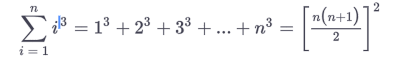than one shows, it holds true for a specific value e.g. 1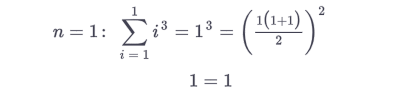Than the "fun" part begins when you show it doesn't matter what value you choose for example, k and k +1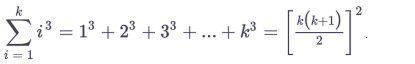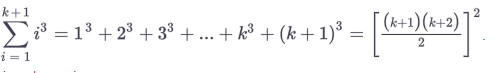than you show that your asumptions hold true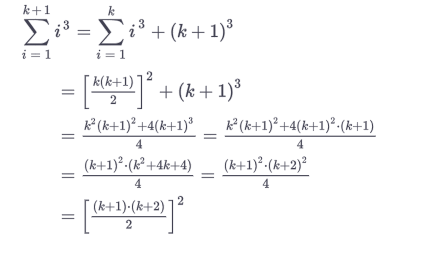and your done, I never wrote anything else extra in my life after that.

or do you mean you had to write down the structure of the proof?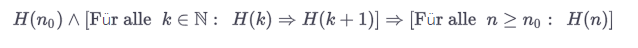sorry for the german text: Für alle = for all = ∀

• @J.Hilk
That is very pretty! But, no, it was not just a question of writing QED. I refer you to the quote I pasted above, where you can see that another person has the same recollection as I have about some very specific wording we were made to use, which we have now forgotten. It was something about what we had to write for the move to generalise from `1`/`k`/`k + 1` to `n`. And something similar in the way of "special wording" when we talked about the confidence-relationship between x-bar & mu in statistics....